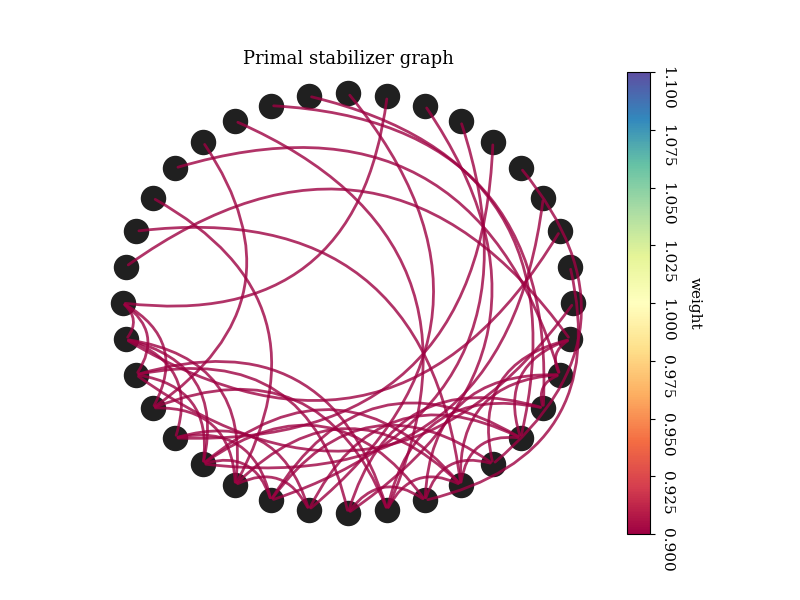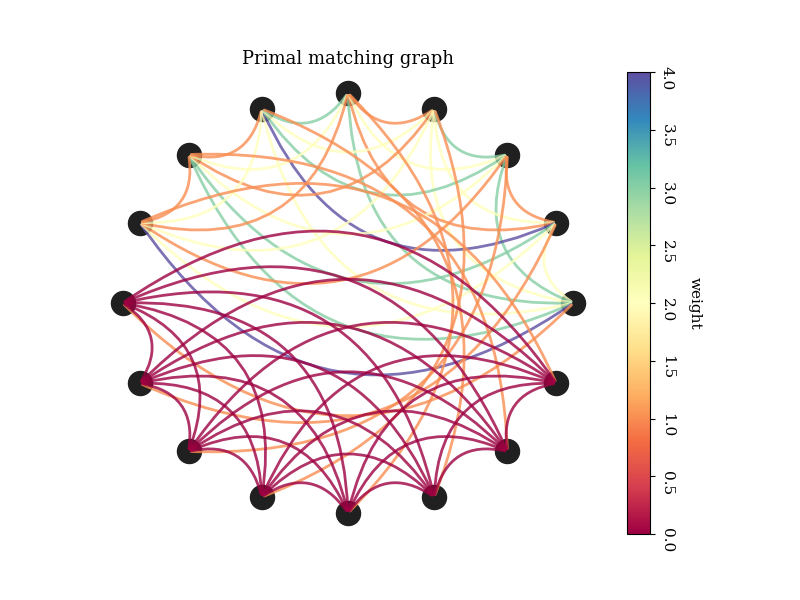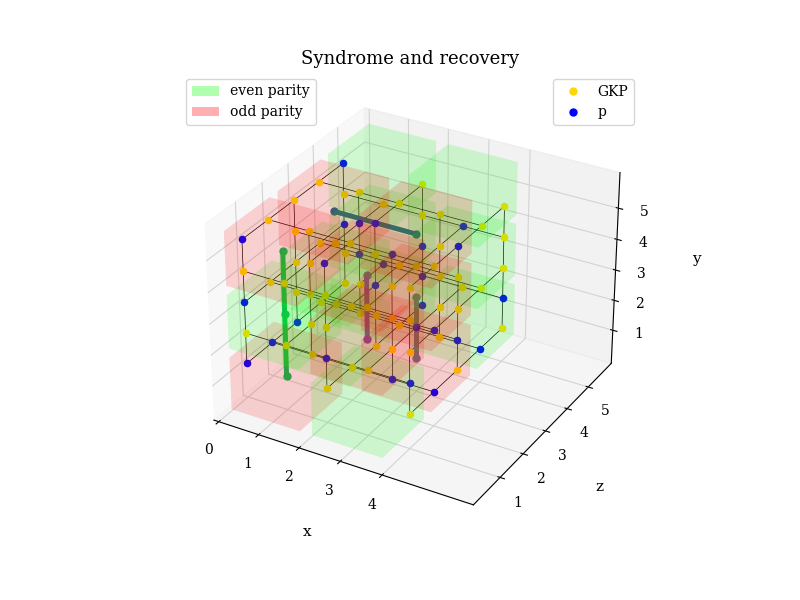# Getting Started¶

There is a vast literature available to understand the theoretical concepts behind FlamingPy. For a self-contained description, see Xanadu’s blueprint for a fault-tolerant photonic quantum computer. To see a sample of what FlamingPy can do, let us first import a few important objects.

from flamingpy.codes import SurfaceCode
from flamingpy.decoders import correct
from flamingpy.noise import CVLayer


Next, let us instantiate an RHG lattice – the measurement-based version of the surface code.

RHG = SurfaceCode(3)


The integer denotes the code distance. By default, the boundaries are set to “open”. Next, let us associate the nodes in the RHG lattice with CV states and assume a noise model. We will choose a Gaussian random noise model in this example. To do so, we will have to specify two parameters: the finite-energy parameter $$\delta$$ and the swap-out probability $$p_{swap}$$ that designates the probability of having a p-squeezed state instead of a GKP qubit. Finally, we simulate a syndrome measurement (sequence of homodyne measurements) on the lattice, with the outcomes translated to bit values.

CVRHG = CVLayer(RHG, delta=0.08, p_swap=0.25)
CVRHG.apply_noise()


At this point, we are ready to perform error correction on the code. We can choose the decoder, but also if (and how) we want to visualize the process. Let’s do so to get a better understanding of what is going on.

# Drawing options
node_colors = ("state", {"GKP": "gold", "p": "blue"})

dw = {
"show_nodes": True,
"color_nodes": node_colors,
"show_recovery": True,
"label_stabilizers": False,
"label_boundary": False,
"label_edges": False,
"label": None,
"legend": True,
"show_title": True,
"show_axes": True,
}

c = correct(RHG, decoder="MWPM", draw=True, drawing_opts=dw)

•••Finally, let’s print a message identifying success or failure.

outcome = "succeeded." * bool(c) + "failed." * (1 - bool(c))
message = "Error correction {}".format(outcome)
print(message)

Error correction failed.


Total running time of the script: ( 0 minutes 2.086 seconds)

Gallery generated by Sphinx-Gallery

Home

Background

Using FlamingPy

Development

Getting Help

Python API